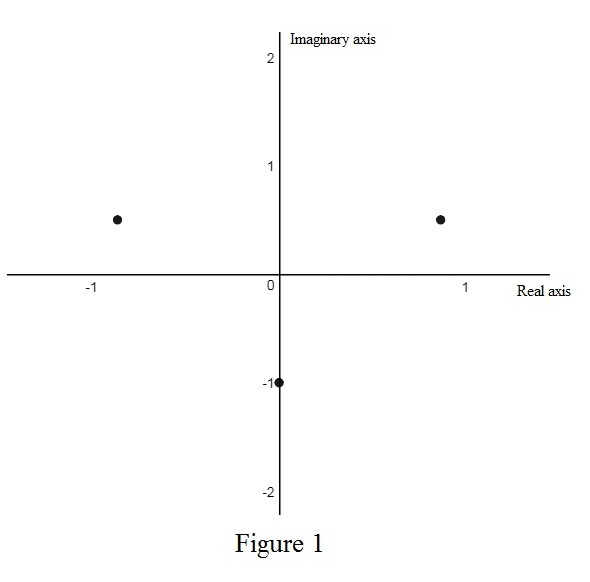# The roots of the cube root of i and sketch the roots in the complex plane.### Single Variable Calculus: Concepts...

4th Edition
James Stewart
Publisher: Cengage Learning
ISBN: 9781337687805### Single Variable Calculus: Concepts...

4th Edition
James Stewart
Publisher: Cengage Learning
ISBN: 9781337687805

#### Solutions

Chapter I, Problem 39E
To determine

## To find: The roots of the cube root of i and sketch the roots in the complex plane.

Expert Solution

The roots of cube root of i are wk=113[cos(π2+2kπ3)+isin(π2+2kπ3)] where k=0,1,2.

### Explanation of Solution

Theorem used:

Roots of a complex number:

Let z=r(cosθ+isinθ) and n be a positive integer. Then z has the n distinct nth roots wk=r1n[cos(θ+2kπn)+isin(θ+2kπn)] where k=0,1,2,,n1.

Calculation:

Rewrite the complex number i in polar form.

The polar form of the complex number z=a+bi is z=r(cosθ+isinθ) where r=|z|=a2+b2  and tanθ=ba.

Consider the complex number i. Here, i=0+i. So, a=0, b=1 and n=3.

Obtain the argument of the complex number i.

tanθ=10θ=tan1(10)θ=π2

Thus, the argument of the complex number i is θ=π2.

Obtain the modulus of the complex number i.

r=|i|=02+(1)2=1=1

Thus, the value of r=1.

Therefore, the polar form of the complex number i is 1=(cosπ2+isinπ2).

By the above theorem, the roots of cube root of i are wk=113[cos(π2+2kπ3)+isin(π2+2kπ3)] where k=0,1,2.

Use online calculator to sketch the roots in the complex plane as shown below in Figure 1.From figure 1, it is observed that all cube root of i form a triangle on complex plane.

### Have a homework question?

Subscribe to bartleby learn! Ask subject matter experts 30 homework questions each month. Plus, you’ll have access to millions of step-by-step textbook answers!